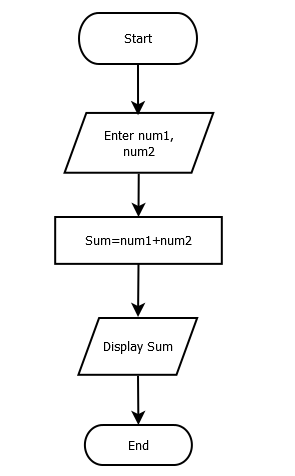# Write a Program to Add Two Numbers

Addition of two numbers is a sum of two numberic values. For example, if we have two values 10 and 20, sum of these values is 30. We can refer Algorithm and flowchart of this program as well for better understanding.

#### Flowchart:#### Algorithm:

Begin:

Step1: Take two numeric values from the user

Step2: Verify both values are numeric

Step3: Add both values and store result into a new variable

Step4: Display the result

End:

#### Program Example

This program is tested and executed using Atom IDE with Python 3.8 version. To add numeric values, we cast user input to int because input function returns string type value. For more details refer this User Input.

x=int(input('Enter first value'))
y=int(input('Enter second value'))
z = x+y
print("sum=",z)
Output:
Enter first value 20
Enter second value 20
sum= 40
Trending